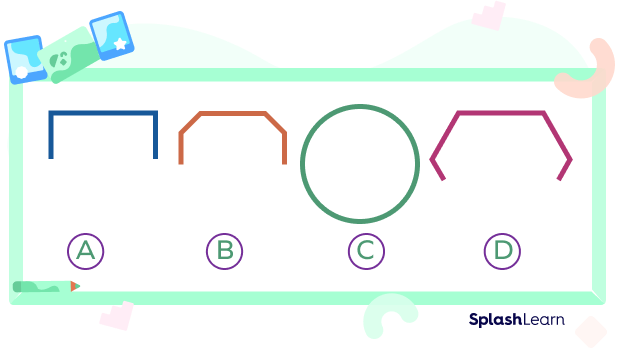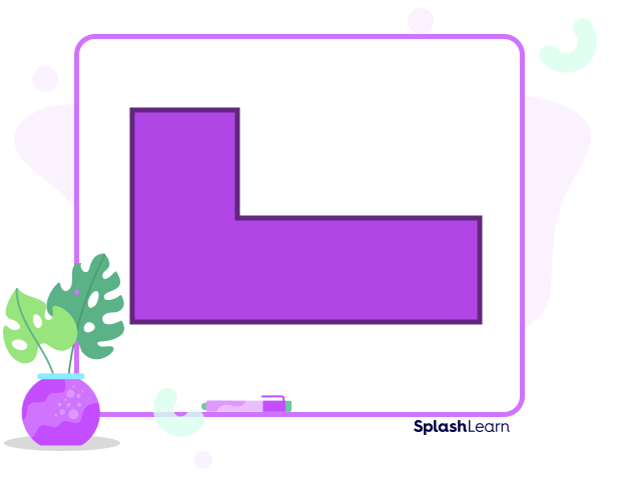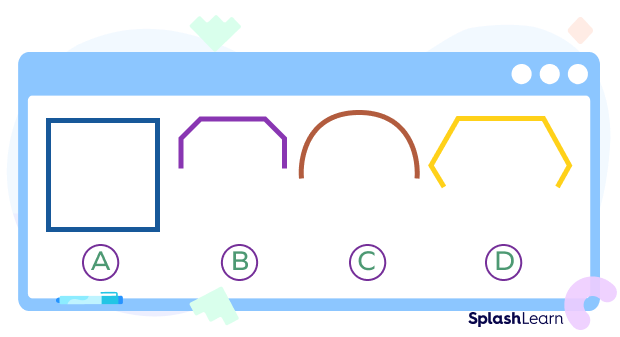# What is Closed Shapes? Definition With Examples

In our day-to-day life, we come in contact with various objects around us. Have you ever thought about their shapes? Why and how does each object have a different shape?

Pick any object near you and closely observe its structure. Start from any one point on the object and see if you can trace its boundary completely and come back to the same point from where you started. This is how we identify closed and open shapes. Let’s look at some examples.

This is a mosquito coil. As you can see, it does not end at the point where it starts, so it is an open shape.

When we draw an open shape, the point where you start will not end at the same point or at the starting point.

Now,  look at the picture of the choco-chip cookie below. Its starting point and ending point are the same, so we can call it a closed shape.

In a closed shape, the endpoint of the shape meets the point where it began.

## What is Closed Shapes?

In geometry, a closed shape can be defined as an enclosed shape or figure whose line segments and/or curves are connected or meet end to end. Closed shapes start and end at the same point.

The least number of sides required to make a closed shape is 3, which forms a polygon named a triangle.

## Difference between Open and Closed Shapes

The following table mentions all the differences between open and closed shapes based on their definitions. Let’s have a look:

## Closed Shapes in Geometry

The least number of sides a closed shape can have is 3, which is a polygon named triangle.

• A triangle is a three-sided closed shape polygon having three vertices.
• A circle is a closed shape with one face and no sides or vertices.
• A quadrilateral is a four-sided closed shape having four vertices. Square, rectangle, rhombus, parallelogram, and trapezium are some examples of quadrilaterals.
• A pentagon is a five-sided closed shape with five vertices.

There are many more closed shapes in geometry than the ones listed above! As a matter of fact, a polygon is always a closed shape.

## Solved Examples

Example 1. How many sides does this shape have? Is the shape closed or open?

Solution: First, we count the number of straight lines in the shape.

There are eight straight lines connected to each other, making an octagon.

As this octagon’s outline may be traced without any gaps, it is a closed shape.

Example 2. Are straight lines necessary to make closed shapes?

Solution: No, straight lines are not necessary to make a closed shape. Some closed shapes that have no straight lines are oval, semicircles, etc. A semicircle is a closed shape with one curved edge and one straight edge.

Example 3. Which of these figures are closed and open shapes?

Alt Tag: Open shapes and closed shapeSolution: Option (C) is a circle with no gaps along its boundary. Hence, it is a closed shape. All the other shapes have gaps in their boundaries and hence, they are open shapes.

## Practice Problems

1

### Which of these is a closed shape?

Shape A
Shape B
Shape C
Shape D
CorrectIncorrect
The circle does not have any gaps along its boundary and hence, it is a closed shape.2

### How many sides does this closed shape have?

6
5
8
5
CorrectIncorrect
This closed shape has 6 sides. It is an irregular hexagon.3

### Which of these is a closed shape?

Shape A
Shape B
Shape C
Shape D
CorrectIncorrect
The first shape does not have any gaps, making it a closed shape. It is a square.4

Arc
Circle
Oval
Semi Circle
CorrectIncorrect